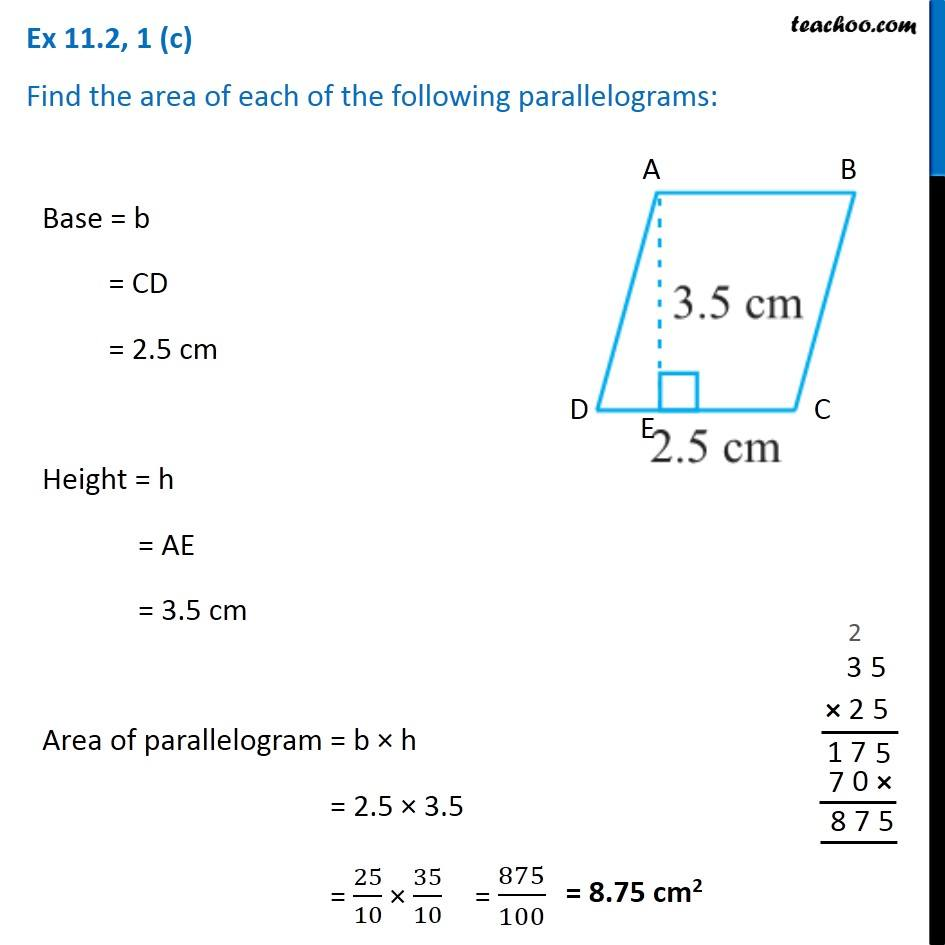Ex 11.2

Chapter 11 Class 7 Perimeter and Area
Serial order wiseGet live Maths 1-on-1 Classs - Class 6 to 12

### Transcript

Ex 11.2, 1 (c) Find the area of each of the following parallelograms: Base = b = CD = 2.5 cm Height = h = AE = 3.5 cm Area of parallelogram = b × h = 2.5 × 3.5 = 25/10 × 35/10